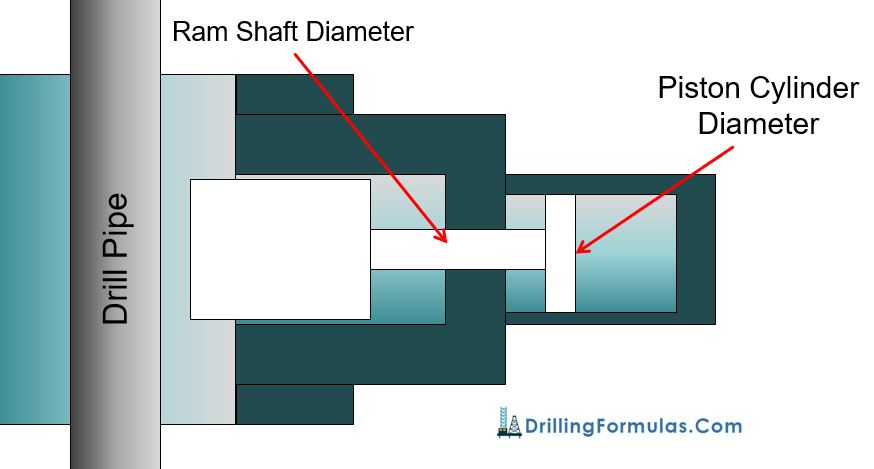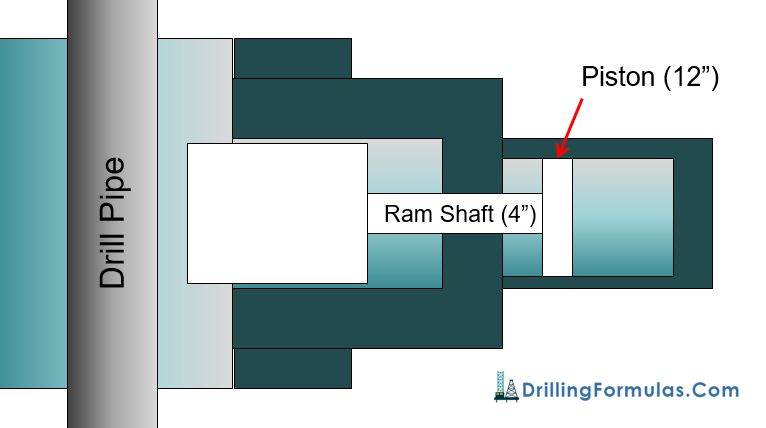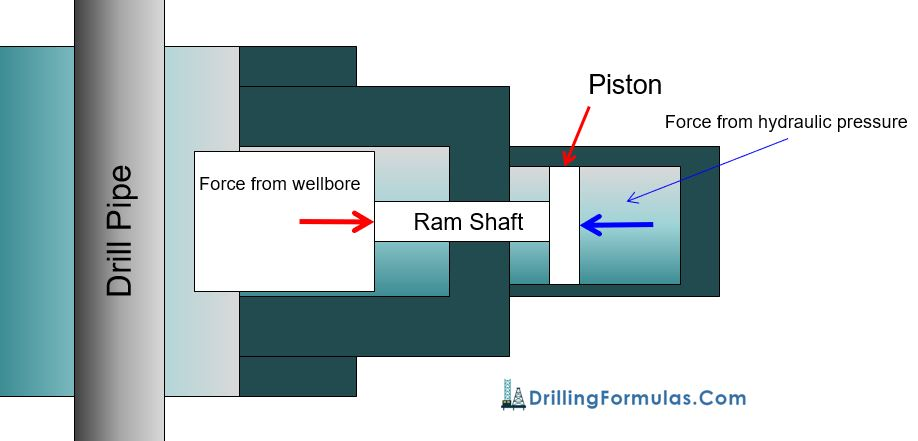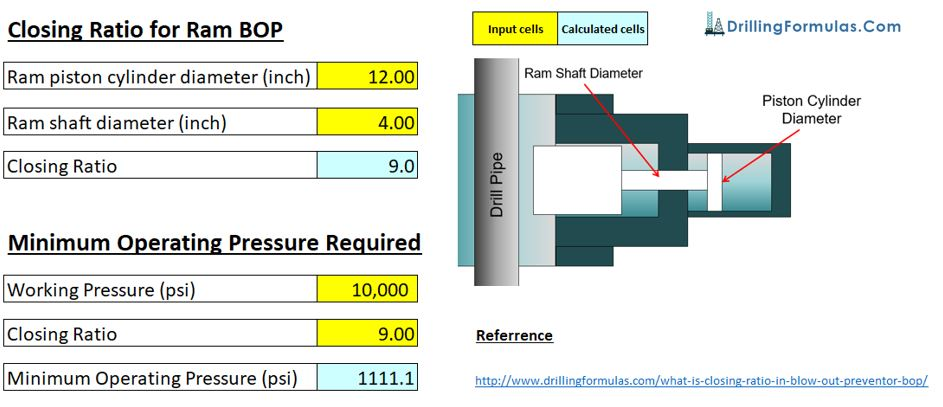# What is Closing Ratio in Blow Out Preventer (BOP)?

People asked me about what the closing ratio is and what it tells us. Closing ratio is defined as the cross sectional area of the ram piston (cylinder) divided by the cross sectional area of the ram shaft. The closing ratio is used to determine Ram closing pressure which will overcome wellbore pressure acting to Ram body. The closing ratio formula is below;

### Closing Ratio = Ram Piston Cylinder Area ÷ Ram Shaft Area

Before going into the detailed calculation, we would like to show you where the cylinder and the ram shaft are in BOP. In Figure 1 (Shaffer SL-Ram BOP), the yellow shaded parts demonstrate these two areas which will be used to calculate the closing ratio.Figure 1 – Shaffer SL-Ram BOP

Detailed Formulas are as follows;

Ram Piston Cylinder Area  (square inch) = ( π× Ram Piston Cylinder Diameter) ÷ 4

Ram Shaft Area  (square inch) = ( π× Ram Shaft Diameter) ÷ 4

Closing Ratio = Ram Piston Cylinder Area ÷ Ram Shaft AreaFigure 2 – Diagram of Closing Ratio Calculation

Example: Ram has a piston cylinder diameter of 12 inch and 4” of ram shaft diameter (see Figure 3).

Ram piston area = (π x 122 ) ÷ 4 = 113.1 square inch

Ram shaft area = (π x 42 ) ÷ 4 = 12.6 square inch

Closing Ratio = 113.1 ÷ 12.6 = 9.0Figure 3 -Basic Diagram of Rams

Figure 2 – Basic Diagram of Rams

### How To Use Closing Ratio To Determine Minimum Operating Pressure

When you know the closing pressure of the BOP ram, you can use the figure to determine the minimum operating pressure. The following equation is used to determine the minimum operating pressure from the accumulator unit (koomey).

### Minimum Operating Pressure = Working Pressure ÷ Closing Ratio

Example: What is the minimum operating pressure would be needed to close the ram against 10,000 psi maximum anticipated pressure on BOP?

For this example, we will use the ram details from the example above.

Minimum Operating Pressure = 10,000 ÷ 9 = 1,111 psi

With operating pressure of 1,111 psi, hydraulic force will be equal to force acting from the wellbore in this case (see Figure 4).Figure 4 – Force Acting at Ram Shaft and Force at Piston

In this case, a standard accumulator (3,000 psi system) with minimum operating pressure of 1,200 psi is good enough to shut the well in with 10,000 psi surface pressure. If you need to handle larger pressure, for instant 15,000 psi, you may need to consider bigger rams which will have larger closing ratio.

Excel Spreadsheet for Closing Ratio Calculation

The calculation sheet for closing ration calculation is provided for you to download for free and this file is unlocked so you can modify for your future work.Figure 5 – Closing Ratio Excel Spreadsheet

Download the spreadsheet >>> Closing Ratio in Blow Out Preventor

References

Cormack, D. (2007). An introduction to well control calculations for drilling operations. 1st ed. Texas: Springer.

Crumpton, H. (2010). Well Control for Completions and Interventions. 1st ed. Texas: Gulf Publishing.

Grace, R. (2003). Blowout and well control handbook [recurso electrónico]. 1st ed. Paises Bajos: Gulf Professional Pub.

Grace, R. and Cudd, B. (1994). Advanced blowout & well control. 1st ed. Houston: Gulf Publishing Company.

Watson, D., Brittenham, T. and Moore, P. (2003). Advanced well control. 1st ed. Richardson, Tex.: Society of Petroleum Engineers.

Share the joyWorking in the oil field and loving to share knowledge.

### 5 Responses to What is Closing Ratio in Blow Out Preventer (BOP)?

1.warren ovie precious says:

Want to know more about drilling and its calculations

2.NM says:

From the equation the pressure from wellbore is acting against only ram shaft area.
It means that the pressure also affects from backside of ram not only in front of ram.
Is there no seal around ram and bop body? (I belive there is a seal on upper side of ram at least)

•DrillingFormulas.Com says:

NM,

Yes. There is only seal on the upperside of BOP rams.

Regards,
Shyne.

3.OPSINGH says:

What is the closing time for rams and annular on a 18 3/4″ BOP with a operating pressure of 1500 psi and where can i find the API recommendation.

•DrillingFormulas.Com says:

Opsingh,

You may need to take a look at API RP53.

This site uses Akismet to reduce spam. Learn how your comment data is processed.